## Organic chemistry - some basic principles and techniques Quiz-18

IIT JEE exam which consists of JEE Main and JEE Advanced is one of the most important entrance exams for engineering aspirants. The exam is held for candidates who are aspiring to pursue a career in the field of engineering and technical studies. Chemistry is important because everything you do is chemistry! Even your body is made of chemicals. Chemical reactions occur when you breathe, eat, or just sit there reading. All matter is made of chemicals, so the importance of chemistry is that it's the study of everything..

Q1.The correct name of the compound (I) is: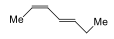•  (E-2), (E-4), Hepta-2,4-diene
•  (Z-2), (Z-4), Hepta-2,4-diene
•  (E-2), (Z-4), Hepta-2,4-diene
•  (Z-2), (E-4), Hepta-2,4-diene
Solution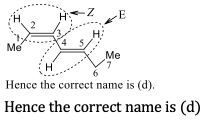Q2.The catalyst used in Kjeldahl's method for the estimation of nitrogen is:
•  Copper
•  Magnesium
•  Mercury
•  Sodium
Solution
CuSO_4 or Hg acts as catalyst

Q3. 0.14 gm of an acid required 12.5 ml of 0.1 N NaOH for complete neturalisation. The equivalent mass of the acid is:
•  63
•  56
•  45
•  112
Solution

Q4. The correct order of acidities of the following is: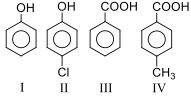•  III>IV>II>I
•  IV>III>I>II
•  III>II>I>IV
•  II>III>IV>I
Solution
III>IV>II>I>(III(acids)>IV(acid with Me)group at p-position (+I and H.C.effect of Me) )>II(Phenol with Cl at p-position (-I effect of Cl) )>I (standard)

Q5. The decreasing order of priority for the following functional groups is: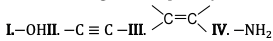•  (IV)> (I)>(II)>(III)
•  IV)> (I)>(III)>(II)
•  (I)> (IV)>(III)>(II)
•  (II)> (III)>(IV)>(I)
Solution

(c)In case of polyfunctional compounds, one of the functional groups is closen as the principal group and the compound is named on that basis. The remaining functional groups, which are subordinate functional groups, are named as substituents using the appropriate prefixes. The decreasing order of priority of some functional groups is -COOH>-SO_3 H>-COOR (ester)>-COCl (acylhalide)>-CONH_2(amide)>-C≡N(nitriles)>-CH=O(aldehyde)> (keto)>-OH(alcohol)>-NH_2(amine)> (alkene)> -C≡C-(alkyne) The–R (alkyl group), Ph or C_6 H_5- (Phenyl), halogens (F,Cl,Br,I),-NO_2, alkoxy(-OR), etc., are always prefix substituents. Thus, a compound containing both an alcohol and a keto group is named hydroxyl alkanone since the keto group is preffered to the hydroxyl group For example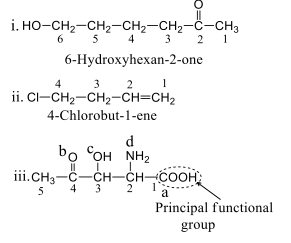(a, b, c, and d are in the decreasing preference order but written in IUPAC name:2-Amino-3-hydroxy-4-oxopentan-1-oic acid
Q6. A compound which does not give a positive test in Lassaigne's test for nitrogen is:
•  Glycine
•  Hydrazine
• Urea
•  Phenyl hydrazine
Solution
Positive Lassiagne's test is given by containing both C and N a. NH_2 CONH_2 (Contains both C and N) b.NH_2-NH_2 (Does not contain C). So, the test is not given by (b) c. Glycine (NH_2-CH_2-COOH).(Contains both C and N) d.Ph–NH— NH_2 (Contains both C and N)

Q7. Which of the following alkenes is most stable?
•  (CH_3 )_2 CH=CH_2
•  CH_3 CH=CHCH_3
•  (CH_3 )_2 C=C(CH_3 )_2
•  CH_3 CH=CH_2
Solution
The most substituted alkene is more stable. Alkene in (c) is most substituted

Q8. The molecular mass of a compound having empirical formula C_2 H_5 O is 90.The molecular formula of the compound is:
•  C_6 H_15 O_3
•  C_4 H_10 O_2
•  C_2 H_5 O
•  C_3 H_6 O_3
Solution

E.F.W.= C_2 H_5 O=12×2+5×16=45 n= (M.W.)/(E.F.W.)=2 Molecular formula =C_4 H_10 O_2

Q9. How many gem dihalides with different formulas are possible for C_3 H_6 CI_2?
•  1
•  2
•  3
•  4
Solution

Q10. n-Butane (C_4 H_10) is produced by monobromination of C_2 H_6 followed by Wurtz reaction. Calculate the volume of ethane at S.T.P. required to produce 55 gm of n-butane. The bromination takes place with 90% yield and the Wurtz reaction with 85% yield
•  27.75 litres
•  55.5 litres
•  55.5 litres
• 5.55 litres
Solution
2C_2 H_6+Br_2→2C_2 H_5 Br+HBr□(→┴( 2Na ) ) C_4 H_10 12×4+10=58 gm 58 gm of n-butane ⇒2×22.4litre of C_2 H_6at STP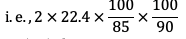=58.56 litre at STP ∴ 58 gm of n-butane =58.56 litre 55 gm of n-butane =55.5 litre#### Written by: AUTHORNAME

AUTHORDESCRIPTION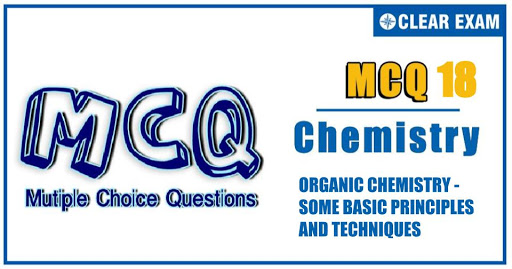## Want to know more

Please fill in the details below:

## Latest NEET Articles\$type=three\$c=3\$author=hide\$comment=hide\$rm=hide\$date=hide\$snippet=hide

Name

ltr
item
BEST NEET COACHING CENTER | BEST IIT JEE COACHING INSTITUTE | BEST NEET, IIT JEE COACHING INSTITUTE: ORGANIC CHEMISTRY - SOME BASIC PRINCIPLES AND TECHNIQUES Quiz-18
ORGANIC CHEMISTRY - SOME BASIC PRINCIPLES AND TECHNIQUES Quiz-18